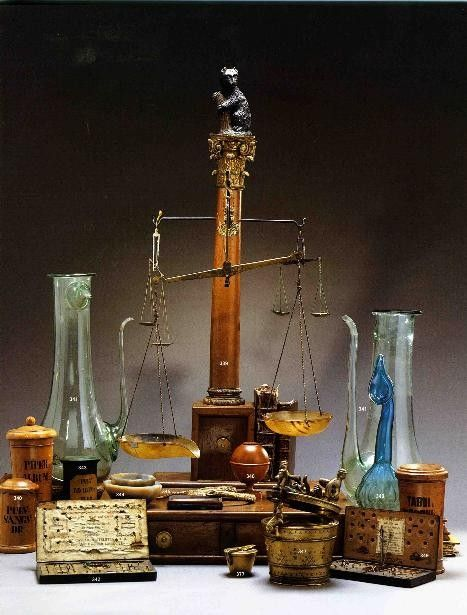Kitchen Equivalents:

• 1 minimum (liquid measure) = 1 drop
• 1 grain (dry measure) = 1 pinch
• 1 dram (liquid or dry measure) = 1 teaspoon
• 2 drams = 1 dessert spoon
• 1/2 ounce = 1 tablespoon
• 1 ounce = 2 tablespoons
• 2 ounces = 1 wineglass
• 4 ounces = 1 teacup
• 8 ounces (dry measure) = 1 (kitchen) cup
• 2 gills (liquid measure) = 1 (kitchen) cup
• 1 pound(l6 ounces, dry measure) = 2 (kitchen) cups
• 1 pint (liquid measure) = 2 (kitchen) cups

Voirdupois Weight: (larger amounts used in cookery)

• 16 ounces = 1 pound

Official Weights and Measures:
NoteOriginally the word “drachm,” the old Greek coin drachma, of Dioscorides’ day, was used in the apothecaries’ weights to distinguish it from the “dram” of the avoirdupois scale. Unfortunately, in these modern times, both are confusingly written. Wherever dram is referred to in this text, the apothecary weight is meant.)

• 20 drops = 1/5 tsp.
• 100 drops = 1 tsp.
• 1/8 fluid ounce = 1 dram = 1/2 tsp. plus 1/8 tsp.
• 1/4 fluid ounce = 2 drams = 1/2 tbsp.
• 1/2 fluid ounce = 4 drams = 1 tbsp.
• 3/4 fluid ounce = 6 drams =1 tbsp. plus 1/2 tsp.
• 1 fluid ounce = 8 drams =2 tbsp.

Apothecaries’ Weight: (small amounts used in medicine)

• 20 grains = 1 scruple = 10 drops
• 1 minum = ½ drop
• 3 scruples = 60 minims (drops, liquid) = 1 dram (drachm)
• 3 scruples = 1 Tbs.
• 8 drams = 1 ounce
• 128 drams = 16 ounces
• 128 drams = 1 pound

Milliliters to Ounces:

• 1 milliliter = 1/30 ounce
• 2 milliliters = 1/16 ounce
• 4 milliliters = 1/8 ounce
• 10 milliliters = 1/3 ounce
• 15 milliliters = 1/2 ounce
Subscribe
If you'd like to stay up to date on everything that is posted here, subscribe via email: